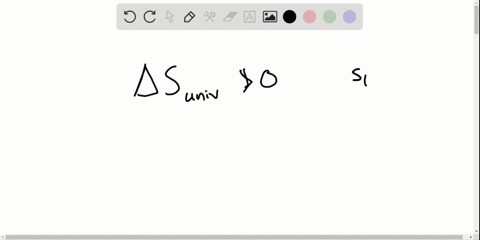Enroll in one of our FREE online STEM summer camps. Space is limited so join now!View Summer Courses### What is the advantage of calculating free energy …

00:42Ohio State University

Need more help? Fill out this quick form to get professional live tutoring.

Get live tutoring
Problem 44

xyacetylene welding is used to repair metal structures, including bridges, buildings, and even the Statue of Liberty. Calculate $\Delta S_{\mathrm{xn}}^{\circ}$ for the combustion of 1 mol of acetylene $\left(\mathrm{C}_{2} \mathrm{H}_{2}\right)$

Check back soon!

## Discussion

You must be signed in to discuss.

## Video Transcript

we want to determine the change in standard Mueller entropy or Delta s reaction for the combustion of one mole of C two h two. We know that for combustion reactions we are reacting in this case C two h two with oxygen in order to produce CO two and water. Since we're interested in the combustion of one mole of C two h two, we could please a one here. But that would lead to us eventually having to use fractions for the other story que metric coefficients. You could do that if you wanted if you would like to, but I personally I like to use whole numbers one balancing the chemical reaction and then dividing out at the end. The final answer. You get the the Delta s of reaction for the combustion of one mole of C two h two. So I'm going to use whole numbers and that would require to put a two there so that we have four carbon atoms on each side of the reaction and that leads to four hydrogen atoms on each side as well. When we place a story key metric coefficients to for water and now we have eight Oxygen's from CO two was to oxygen's from water. So a total of 10 oxygen's on the products that we need to strike you metric coefficient of five here to balance that out. And so now, in order to determine Delta s reaction, we need to find the total entropy of the products and subtract the total entropy of the reactant. So starting with the products, we see that we have four moles of CO two in two moles of H 20 and that corresponds to the end. In this equation, the number of moles in the story geometrically balanced equation. And again, that's four moles of CO two in two moles of H 20 We look up each one of these chemical species standard Moeller entropy values in the appendix and multiply it by the number of moles. And when we do that, the most cancel out to yield units of jewels per kelvin. We're total entropy of the products that comes out to this value. And now, on the reactant side, we see that we have two moles of C two, h two and five moles of 02 So two moles of C two H two and five moles of 02 multiply each one by the respective standard Moeller entropy values to get units of total entropy for the reactant. And now we do products minus reactions. This is the total entropy of the products. This is a total entropy of the reactant. And after we subtracted to we get this answer for Delta s of reaction, I remember because we used whole numbers. We have to divide this answer by two so that when we divide this sticky metric coefficient by two and all the other ones, we get the combustion of one mole of C two h two. And that's what we're interested in. The delta s of reaction for the combustion of one mole of C two h two. So after we divide that answer by two, we get a final value for a delta s of reaction or the combustion of one mole of the ceiling to be negative. 97.2 Jules her Kelvin. And that is the final answer